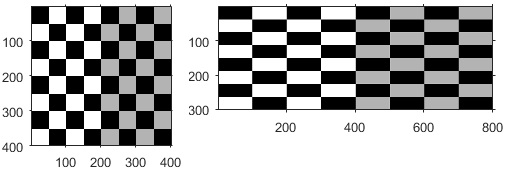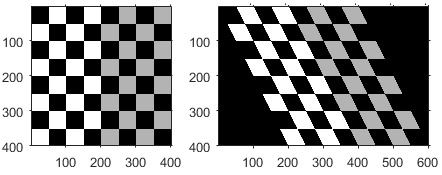## Matrix Representation of Geometric Transformations

You can represent a linear geometric transformation as a numeric matrix. Each type of transformation, such as translation, scaling, rotation, and reflection, is defined using a matrix whose elements follow a specific pattern. You can combine multiple transformations by taking a composite of the matrices representing the transformations. For more information, see Create Composite 2-D Affine Transformations.

### 2-D Affine Transformations

The table lists 2-D affine transformations with the transformation matrix used to define them. For 2-D affine transformations, the last row must be ```[0 0 1]```.

• Use combinations of 2-D translation matrices to create a `transltform2d` object representing a translation transformation.

• Use combinations of 2-D translation and rotation matrices to create a `rigidtform2d` object representing a nonreflective rigid transformation.

• Use combinations of 2-D translation, rotation, and scaling matrices to create a `simtform2d` object representing a nonreflective similarity transformation.

• Use any combination of 2-D transformation matrices to create an `affinetform2d` object representing a general affine transformation.

2-D Affine TransformationExample (Original and Transformed Image)Transformation Matrix
Translation$\left[\begin{array}{ccc}1& 0& {t}_{x}\\ 0& 1& {t}_{y}\\ 0& 0& 1\end{array}\right]$

tx specifies the displacement along the x axis

ty specifies the displacement along the y axis.

Scale$\left[\begin{array}{ccc}{s}_{x}& 0& 0\\ 0& {s}_{y}& 0\\ 0& 0& 1\end{array}\right]$

sx specifies the scale factor along the x axis

sy specifies the scale factor along the y axis.

Shear$\left[\begin{array}{ccc}1& s{h}_{x}& 0\\ s{h}_{y}& 1& 0\\ 0& 0& 1\end{array}\right]$

shx specifies the shear factor along the x axis.

shy specifies the shear factor along the y axis.

Reflection$\left[\begin{array}{ccc}\text{cosd(2}\phi \text{)}& \text{sind(2}\phi \text{)}& 0\\ \text{sind(2}\phi \text{)}& -\text{cosd(2}\phi \text{)}& 0\\ 0& 0& 1\end{array}\right]$

φ specifies the angle of the axis of reflection, in degrees.

Two common reflections are vertical and horizontal reflection. Vertical reflection is reflection about the x-axis, so φ is 0 and the reflection matrix simplifies to:

`[1 0 0; 0 -1 0; 0 0 1]`.

Horizontal reflection is reflection about the y-axis, so φ is 90 and the reflection matrix simplifies to:

`[-1 0 0; 0 1 0; 0 0 1]`

Rotation$\left[\begin{array}{ccc}\mathrm{cosd}\left(\text{θ}\right)& -\mathrm{sind}\left(\text{θ}\right)& 0\\ \mathrm{sind}\left(\text{θ}\right)& \mathrm{cosd}\left(\text{θ}\right)& 0\\ 0& 0& 1\end{array}\right]$

θ specifies the angle of rotation about the origin, in degrees.

### 2-D Projective Transformations

Projective transformation enables the plane of the image to tilt. Parallel lines can converge towards a vanishing point, creating the appearance of depth.

The transformation is a 3-by-3 matrix. Unlike affine transformations, there are no restrictions on the last row of the transformation matrix. Use any composition of 2-D affine and projective transformation matrices to create a `projtform2d` object representing a general projective transformation.

2-D Projective Transformation ExampleTransformation Matrix
Tilt`$\left[\begin{array}{ccc}1& 0& 0\\ 0& 1& 0\\ E& F& 1\end{array}\right]$`

E and F influence the vanishing point.

When E and F are large, the vanishing point comes closer to the origin and thus parallel lines appear to converge more quickly.

### 3-D Affine Transformations

The table lists the 3-D affine transformations with the transformation matrix used to define them. Note that in the 3-D case, there are multiple matrices, depending on how you want to rotate or shear the image. For 3-D affine transformations, the last row must be `[0 0 0 1]`.

• Use combinations of 3-D translation matrices to create a `transltform3d` object representing a translation transformation.

• Use combinations of 3-D translation and rotation matrices to create a `rigidtform3d` object representing a nonreflective rigid transformation.

• Use combinations of 3-D translation, rotation, and scaling matrices to create a `simtform3d` object representing a nonreflective similarity transformation.

• Use any combination of 3-D transformation matrices to create an `affinetform3d` object representing a general affine transformation.

3-D Affine TransformationTransformation Matrix
Translation

Translation by amount tx, ty, and tz in the x, y, and z directions, respectively:

`$\left[\begin{array}{cccc}1& 0& 0& {t}_{x}\\ 0& 1& 0& {t}_{y}\\ 0& 0& 1& {t}_{z}\\ 0& 0& 0& 1\end{array}\right]$`

Scale

Scale by scale factor sx, sy, and sz in the x, y, and z dimensions, respectively:

`$\left[\begin{array}{cccc}{s}_{x}& 0& 0& 0\\ 0& {s}_{y}& 0& 0\\ 0& 0& {s}_{z}& 0\\ 0& 0& 0& 1\end{array}\right]$`

Shear

Shear within the y-z plane:

`$\left[\begin{array}{cccc}1& 0& 0& 0\\ s{h}_{xy}& 1& 0& 0\\ s{h}_{xz}& 0& 1& 0\\ 0& 0& 0& 1\end{array}\right]$`

such that

`$\begin{array}{l}x\text{'}=x\\ y\text{'}=y+s{h}_{xy}x\\ z\text{'}=z+s{h}_{xz}x\end{array}$`

Shear within the x-z plane:

`$\left[\begin{array}{cccc}1& s{h}_{yx}& 0& 0\\ 0& 1& 0& 0\\ 0& s{h}_{yz}& 1& 0\\ 0& 0& 0& 1\end{array}\right]$`

such that

`$\begin{array}{l}x\text{'}=x+s{h}_{yx}y\\ y\text{'}=y\\ z\text{'}=z+s{h}_{yz}y\end{array}$`

Shear within the x-y plane:

`$\left[\begin{array}{cccc}1& 0& s{h}_{zx}& 0\\ 0& 1& s{h}_{zy}& 0\\ 0& 0& 1& 0\\ 0& 0& 0& 1\end{array}\right]$`

such that

`$\begin{array}{l}x\text{'}=x+s{h}_{zx}z\\ y\text{'}=y+s{h}_{zy}z\\ z\text{'}=z\end{array}$`

Reflection

Reflection across the y-z plane, negating the x coordinate:

`$\left[\begin{array}{cccc}-1& 0& 0& 0\\ 0& 1& 0& 0\\ 0& 0& 1& 0\\ 0& 0& 0& 1\end{array}\right]$`

Reflection across the x-z plane, negating the y coordinate:

`$\left[\begin{array}{cccc}1& 0& 0& 0\\ 0& -1& 0& 0\\ 0& 0& 1& 0\\ 0& 0& 0& 1\end{array}\right]$`

Reflection across the x-y plane, negating the z coordinate:

`$\left[\begin{array}{cccc}1& 0& 0& 0\\ 0& 1& 0& 0\\ 0& 0& -1& 0\\ 0& 0& 0& 1\end{array}\right]$`

Rotation

Rotation within the y-z plane, by angle θx about the x axis, in degrees:

`$\left[\begin{array}{cccc}1& 0& 0& 0\\ 0& \text{cosd}\left({\theta }_{x}\right)& -\text{sind}\left({\theta }_{x}\right)& 0\\ 0& \text{sind}\left({\theta }_{x}\right)& \text{cosd}\left({\theta }_{x}\right)& 0\\ 0& 0& 0& 1\end{array}\right]$`

Rotation within the x-z plane, by angle θy about the y axis, in degrees:

`$\left[\begin{array}{cccc}\text{cosd}\left({\theta }_{y}\right)& 0& \text{sind}\left({\theta }_{y}\right)& 0\\ 0& 1& 0& 0\\ -\mathrm{sind}\left({\theta }_{y}\right)& 0& \text{cosd}\left({\theta }_{y}\right)& 0\\ 0& 0& 0& 1\end{array}\right]$`

Rotation within the x-y plane, by angle θz about the z axis, in degrees:

`$\left[\begin{array}{cccc}\text{cosd}\left({\theta }_{z}\right)& -\text{sind}\left({\theta }_{z}\right)& 0& 0\\ \text{sind}\left({\theta }_{z}\right)& \text{cosd}\left({\theta }_{z}\right)& 0& 0\\ 0& 0& 1& 0\\ 0& 0& 0& 1\end{array}\right]$`

### 3-D Projective and N-D Transformations

The `imwarp` function does not support 3-D projective transformations or N-D affine and projective transformations. Instead, you can create a spatial transformation structure from a geometric transformation matrix using the `maketform` function. Then, apply the transformation to an image using the `tformarray` function. For more information, see N-Dimensional Spatial Transformations.

The dimensions of the transformation matrix must be (N+1)-by-(N+1). The `maketform` and `tformarray` functions use the postmultiply matrix convention. Geometric transformation matrices in the postmultiply convention are the transpose of matrices in the premultiply convention. Therefore, for N-D affine transformation matrices, the last column must contain ```[zeros(N,1); 1]``` and there are no restrictions on the values of the last row.